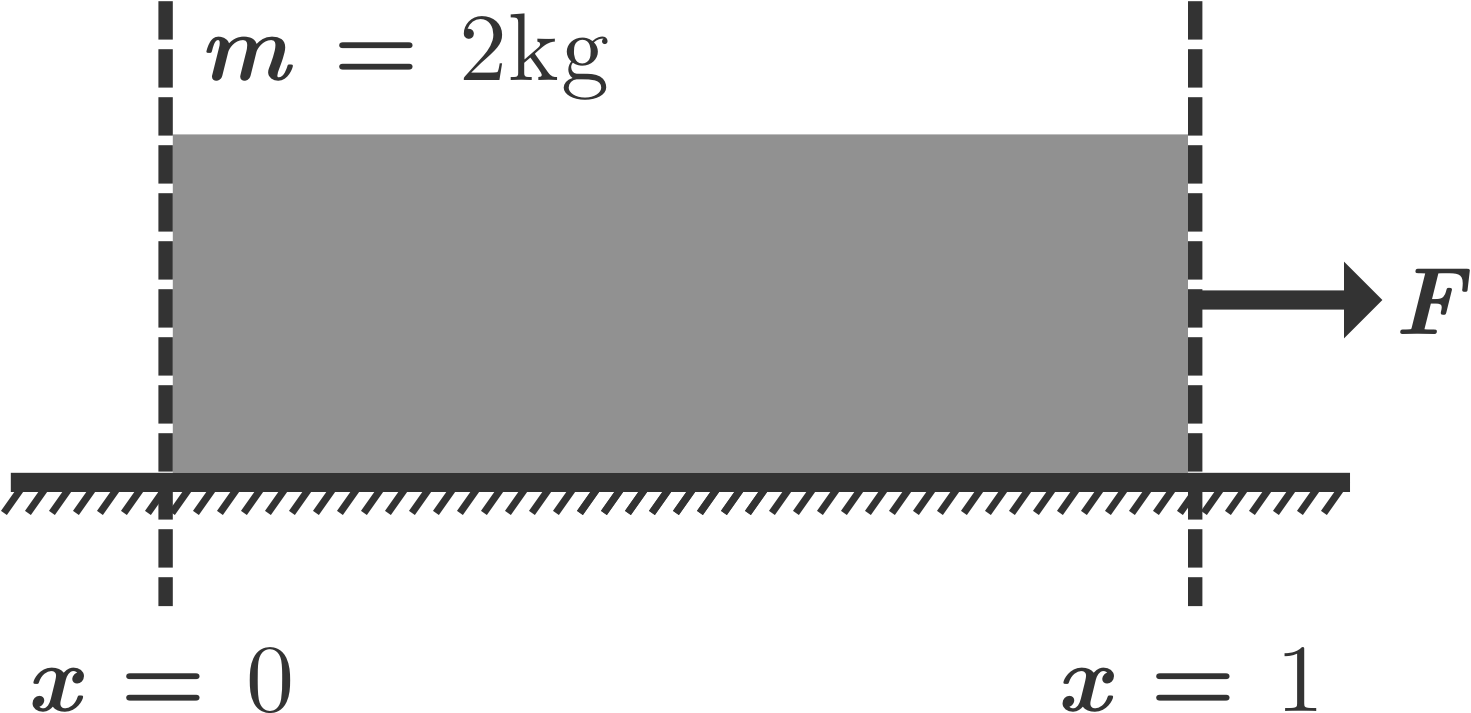# Variable friction Work DoneA block of mass $2 \text{ kg}$ and length $1 \text{ m}$ is placed on a rough surface (having variable kinetic friction coefficient). A horizontal force $F$ is applied such that block moves slowly. If total heat lost in this process is $\frac{4N}{3}$ Joule. Find the value of '$N$'.

Assume $g = 10 \text{ m/s}^2$, ($\mu_k = x$ for $0 \leq x \leq 1$ & $\mu_k = 0$ for $x \geq 1$)

×